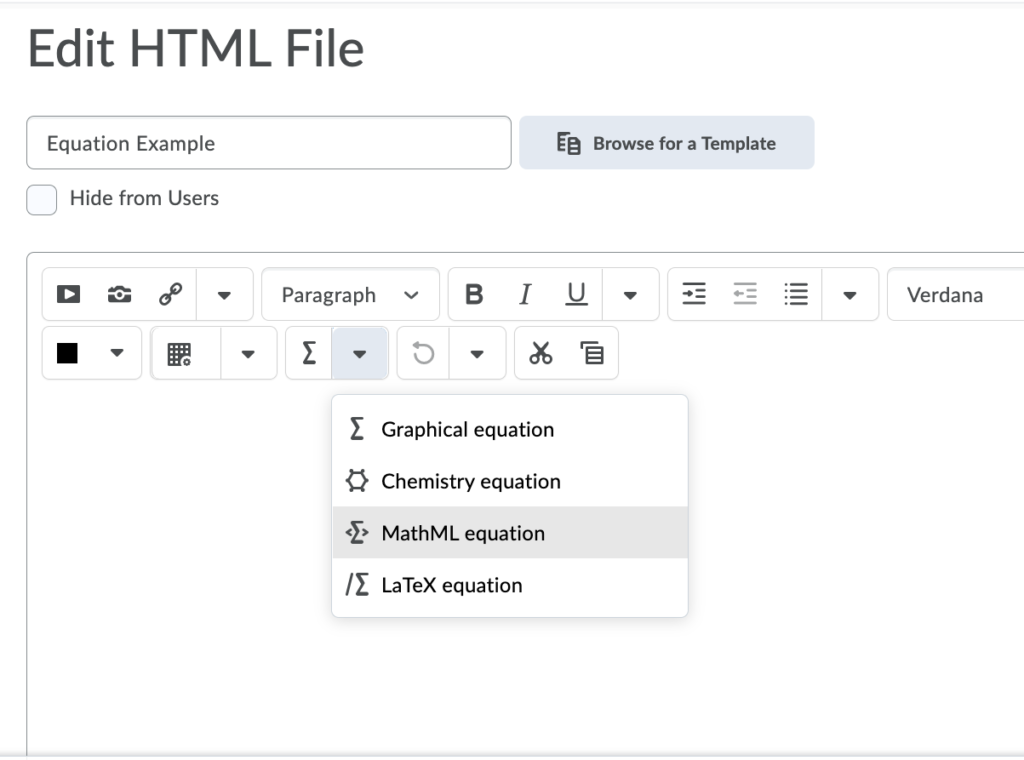# 7 Formulas

In this section, we review how to add accessible formulas to content.

# What are formulas?

Formulas refer to math equations and science formulas.

File types used:  LaTex, MathType

Note: Both of these types of files are available in VIULearn

# Who are you doing this for?

This work supports students who:

• Are blind or have low vision
• Have a form of cognitive disability
• Have a physical disability

# What do you need to do?

There are several ways to handle equations, from images with alt tags to MathML. MathML is available in the HTML text editor in VIULearn,

# MathML

Math ML is a text-based XML (short for “extensible markup language”) designed for math equations. Browsers that support MathML are able to translate the XML into a formatted equation. Since MathML with MathJax can be rendered in many systems, including HTML, sites at Penn State, Angel and Drupal, it is considered the best choice for accessibility.

Here is information about creating and viewing MathML.

MathML may vary from system to system and the content can change rapidly.

MathML is available via the Graphical equation menu in VIULearn. The screenshot below shows where to find it.# Image with an alt tag

A safe option is to create an image of an equation (or export it from an equation editor) and then insert the image into a document with an alt tag.

### Example 1: An equation in HTML

View the alt tag

alt = “m equals begin fraction m sub 0 over begin square root 1 minus begin fraction v sup 2 over c sup 2 end fraction end square root end fraction”

# LaTeX

LaTeX is a math markup language familiar to many in the science and math community. Unfortunately, it is not currently supported by screen reader technology. It is, however, fairly simple to convert LaTeX to an image or MathML in most equation editors.

To import LaTeX, follow these steps in MathMagic and MathType:

1. Copy a piece of LaTeX code such as
 m &= \frac{m_0}{\sqrt{1-\frac{v^2}{c^2}}}
into an equation editor’s main editing window.
2. The equation should appear fully formatted. Make minor adjustments as needed.

At a BCcampus user-testing session, students indicated that it would be helpful to place an audio file of the formula or equation alongside each, allowing the user to hear exactly how the formula or equation should be interpreted.

## Math accessibility at Portland Community College

In 2012, Portland Community College departments took a closer look at making math accessible to blind students. Read more about the math accessibility study.

Watch Math Accessibility at Portland Community College. (This video is an open educational resource.)